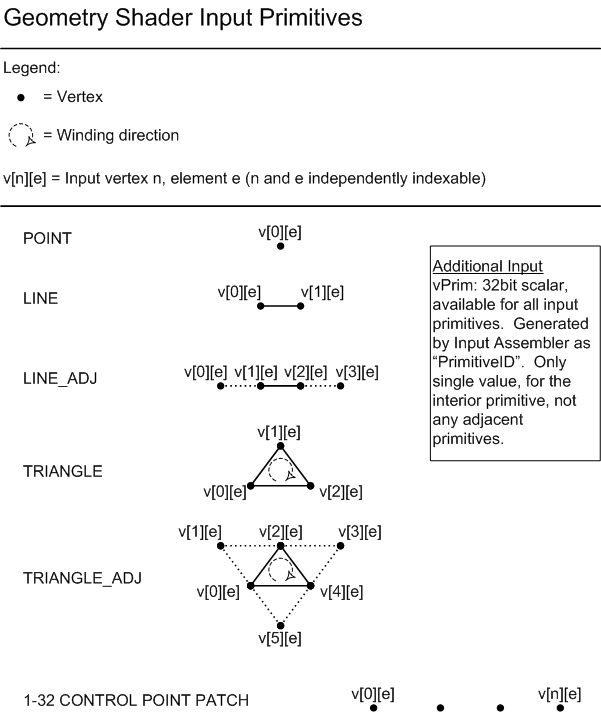A geometry-shader object processes entire primitives. Use the following syntax to declare a geometry-shader object.

[maxvertexcount(NumVerts)] void ShaderName ( PrimitiveType DataType Name [ NumElements ], inout StreamOutputObject );

## Parameters

[maxvertexcount(NumVerts)]

[in] Declaration for the maximum number of vertices to create.

• [maxvertexcount()] - required keyword; brackets and parenthesis are required characters for correct syntax.
• NumVerts - An integer number representing the number of vertices.

[in] An ASCII string that contains a unique name for the geometry-shader function.

PrimitiveType DataType Name [ NumElements ]

PrimitiveType - Primitive type, which determines the order of the primitive data.

Primitive Type Description
point Point list
line Line list or line strip
triangle Triangle list or triangle strip

DataType - [in] An input data type; can be any HLSL data type.

Name - Argument name; this is an ASCII string.

NumElements - Array size of the input, which depends on the PrimitiveType as shown in the following table.

Primitive Type NumElements
point 
You operate on only one point at a time.
line 
A line requires two vertices.
triangle 
A triangle requires three vertices.
A lineadj has two ends; therefore, it requires four vertices.
A triangleadj borders three more triangles; therefore, it requires six vertices.

StreamOutputObject

The declaration of the stream-output object.

None

## Remarks

The following diagram shows the various primitive types for a geometry shader object.The following diagram shows geometry shader invocations.## Examples

This example is from exercise 1 from the Direct3D 10 Shader Model 4.0 Workshop.

``````[maxvertexcount(3)]
void GSScene( triangleadj GSSceneIn input, inout TriangleStream<PSSceneIn> OutputStream )
{
PSSceneIn output = (PSSceneIn)0;

for( uint i=0; i<6; i+=2 )
{
output.Pos = input[i].Pos;
output.Norm = input[i].Norm;
output.Tex = input[i].Tex;

OutputStream.Append( output );
}

OutputStream.RestartStrip();
}
``````